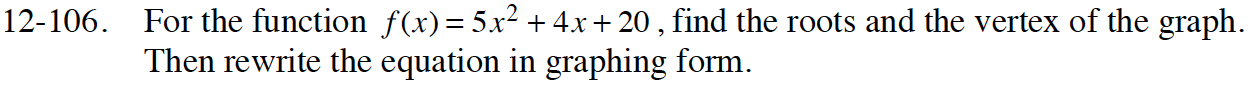Home > CCA2 > Chapter 12 > Lesson 12.2.1 > Problem12-106

12-106.

For the function f(x) = 5x2 + 4x + 20, find the roots and the vertex of the graph. Then rewrite the equation in graphing form. Homework Help ✎Find the roots by using the Quadratic Formula.
Factor and complete the square OR average the intercepts and use substitution to find the vertex.